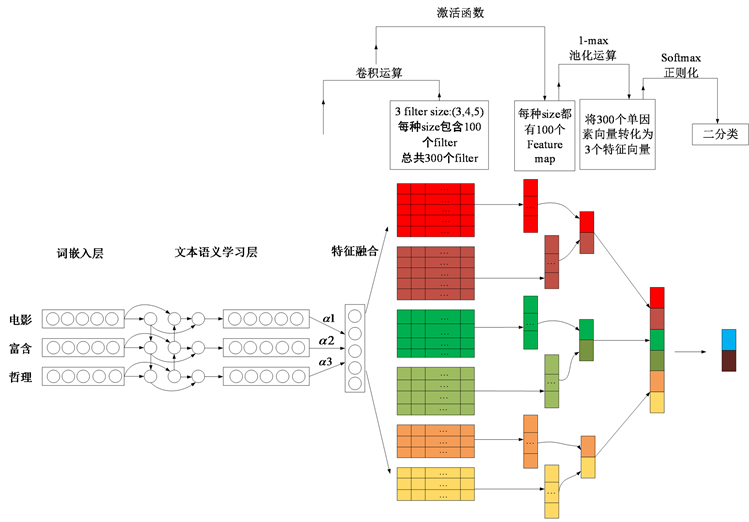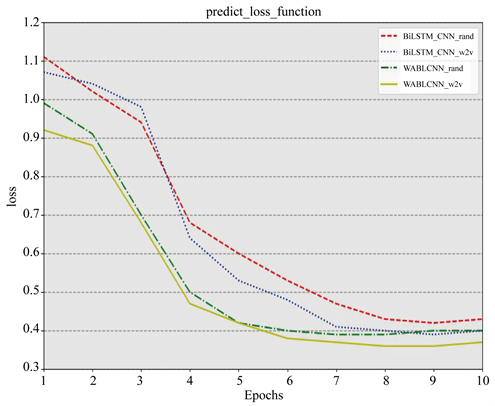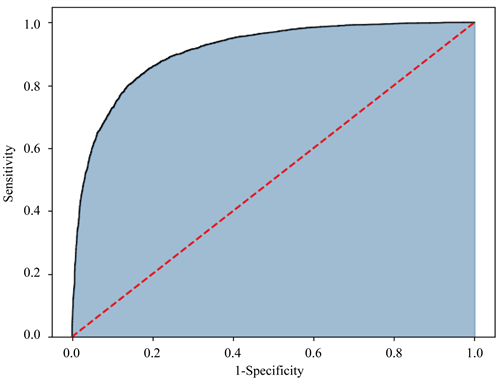# 基于词注意力的BiLSTM和CNN集成模型的中文情感分析Word Attention-Based BiLSTM and CNN Ensemble for Chinese Sentiment Analysis

DOI: 10.12677/CSA.2020.102032, PDF, HTML, XML, 下载: 423  浏览: 1,530

Abstract: Film comments as an important way of expressing emotions in audience viewing, and an im-portant field of emotional mining research. This paper proposes a stacking model based on word attention mechanism BiLSTM and CNN (Word Attention-Based BiLSTM and CNN Stacking Model, WABCSM), and uses the Chinese commentary of the Douban platform as the research object to analyze the emotional tendency of fans to the film. The feasibility of the model. Firstly, the word vector is used to train the text, and the word vector representation is obtained. Then, the fusion model based on word attention mechanism BiLSTM and CNN is used to perform emotion mining to better extract the emotional words in the text to achieve the purpose of correct classification. The model of this paper is valid. Compared with the traditional LSTM model and CNN model, the experimental results show that the classification accuracy and recall are improved. In particular, the addition of the attention mechanism can make the model effectively extract the emotional features, thus effectively overcoming the difficult problem of colloquial short text emotional polarity judgment.

1. 引言

2. 相关工作

3. 基于词注意力机制的BiLSTM和CNN集成模型的中文情感分析Figure 1. Integration model architecture

3.1. 词嵌入层

$S=\left[{x}_{1};{x}_{2};\cdots ;{x}_{L}\right]\in {R}^{L×d}$ (1)

$\begin{array}{l}\epsilon =0\\ \text{FOR}\begin{array}{cc}& u\in Context\left(w\right)\end{array}\begin{array}{cc}& \text{DO}\end{array}\\ \left\{\\ \begin{array}{cc}& \text{FOR}\begin{array}{cc}& j=2:{l}^{u}\end{array}\end{array}\begin{array}{cc}& \text{DO}\end{array}\\ \begin{array}{cc}& \begin{array}{l}\left\{\\ \begin{array}{cc}& \begin{array}{l}q=\sigma \left(v{\left(w\right)}^{\text{T}}{\theta }_{j-1}^{u}\right)\\ g=\eta \left(1-{d}_{j}^{u}-q\right)\\ {\theta }_{j-1}^{u}:={\theta }_{j-1}^{u}+gv\left(w\right)\\ e:=e+g{\theta }_{j-1}^{u}\end{array}\end{array}\\ \right\}\end{array}\end{array}\\ \right\}\\ v\left(w\right):=v\left(w\right)+e\end{array}$

3.2. 文本语义学习层

BiLSTM层。循环神经网络(Recurrent neural network, RNN)是传统神经网络的变体，神经元的设计使得网络具有“记忆性”，所以RNN是深度学习的一种常用框架。对于短文本的处理上RNN具有良好的性质，但对于长文本，RNN网络的“记忆”功能越来越差。为了解决这个问题，Hochreiter & Schmidhuber于1997年提出了长短时记忆网络(Long Short-Term Memory, LSTM) 。本文利用的BiLSTM模型是其变种，为双向长短时记忆网络，因为情感词不仅和之前出现的文本相关，还会和后面的文本相关。

LSTM单元结构的设计体现在3个“门”结构上，这三个“门”结构使得LSTM可以实现对历史信息的重要部分的记忆，这体现在网络中的单元(cell)状态的不断更新。首先记t (当前)时刻的候选单元值为 ${\stackrel{¨}{c}}_{t}$，输入值的权重为 ${w}_{xc}$ 以及 $t-1$ 时刻cell输出的权重为 ${w}_{hc}$，则t时刻的候选单元值为

${\stackrel{¨}{c}}_{t}=\mathrm{tanh}\left({w}_{xc}{x}_{t}+{w}_{hc}{h}_{t-1}+{b}_{c}\right)$ (2)

${i}_{t}=\sigma \left(\left[{w}_{xi},{w}_{hi},{w}_{ci},1\right]{\left[{x}_{t},{h}_{t-1},{c}_{t-1},{b}_{i}\right]}^{\text{T}}\right)$ (3)

${f}_{t}=\sigma \left(\left[{w}_{xf},{w}_{hf},{w}_{cf},1\right]{\left[{x}_{t},{h}_{t-1},{c}_{t-1},{b}_{f}\right]}^{\text{T}}\right)$ (4)

${c}_{t}={f}_{t}\otimes {c}_{t-1}+{i}_{t}\otimes {\stackrel{¨}{c}}_{t}$ (5)

${o}_{t}=\sigma \left(\left[{w}_{xo},{w}_{ho},{w}_{co},1\right]{\left[{x}_{t},{h}_{t-1},{c}_{t-1},{b}_{o}\right]}^{\text{T}}\right)$ (6)

${h}_{t}={o}_{t}\otimes \mathrm{tanh}\left({c}_{t}\right)$ (7)

$\stackrel{\to }{{h}_{t}}=\stackrel{\to }{LSTM}\left({h}_{t-1},{x}_{t},{c}_{t-1}\right)$

$\stackrel{←}{{h}_{t}}=\stackrel{←}{LSTM}\left({h}_{t+1},{x}_{t},{c}_{t+1}\right)$

${h}_{t}=\left[\stackrel{\to }{{h}_{t}},\stackrel{←}{{h}_{t}}\right]$

$T=\mathrm{tanh}\left(Wx+b\right)$ (8)

${\alpha }_{i}=\frac{\mathrm{exp}\left(T\right)}{{\sum }_{i=1}^{S}\mathrm{exp}\left(T\right)}$ (9)

$\tau =\underset{i=1}{\overset{t}{\sum }}{\alpha }_{i}{x}_{i}$ (10)

3.3. CNN层

${c}_{i}=f\left(w\cdot {{x}^{\prime }}_{i:i+n-1}+b\right)$ (15)

${p}_{i}=\mathrm{max}\left(C\right)$ (16)

$p\left(y|P,{w}_{s},{b}_{s}\right)=\text{softmax}\left(P\cdot {w}_{s}+{b}_{s}\right)$ (17)

4. 实验

4.1. 实验环境

4.2. 实验数据Table 2. Comparison of negative text and positive text

(1) 评论文本和标签的获取；

(2) 去除评论文本标点符号及分词处理；

(3) 将处理后的文本构建字典，并将字典中的词进行token转化，提取出现频数大于5的词来创建词典；

(4) 词向量训练，将句子中存在而字典中不存在的词用随机值代替词向量；

(5) 将数据打乱，用于模型训练。

4.3. 性能评价指标

TP表示正类预测为正类，TN表示负类预测为负类，FP表示负类预测为正类，FN为正类预测为负类。对于模型好坏的评价方式一般采用精准率(Precition)、召回率(Recall)和F1值。

$\text{P}=\frac{\text{TP}}{\text{TP}+\text{FP}}$

$\text{R}=\frac{\text{TP}}{\text{TP}+\text{FN}}$

F1值：

$\text{F}1=\frac{\text{2TP}}{\text{2TP}+\text{FP}+\text{FN}}$

4.4. 实验对比及分析

(1) 基于随机向量的LSTM模型。本模型仅利用随机化的向量来作为样本输入，用于LSTM模型训练。

(2) 基于词向量的LSTM模型。本模型利用word2vec对文本训练词向量作为输入，用于LSTM模型训练。

(3) 基于随机向量的CNN模型。本模型仅利用随机化向量来作为样本输入，用于CNN模型训练。

(4) 基于词向量训练的CNN模型。本模型利用word2vec对文本训练词向量并作为输入，用于CNN模型训练。

(5) 基于随机向量的BiLSTM_CNN模型。该模型是对两种单模型的Stacking融合，将样本的随机向量作为模型的输入。

(6) 基于词向量的BiLSTM_CNN模型。该模型是将样本进行word2vec训练并作为输入。结合BiLSTM_CNN模型进行训练。

(7) 本文模型。基于随机向量作为输入，并加入词注意力机制的BiLSTM_CNN (rand & Word Attention-based BiLSTM & CNN Stacking Model, WABCSM + rand)。

(8) 本文模型。基于词向量训练作为输入，并加入词注意力机制的BiLSTM_CNN (word2vec & Word Attention-based BiLSTM & CNN Stacking Model, WABCSM + w2v)。Table 6. Forecast results (partial)Figure 2. Comparison of changes in loss functionFigure 3. ROC curve of WABCSM model

5. 结论

  周纯洁, 黎巎, 徐翼龙, 等, 文本情感分析研究[J]. 计算机科学, 2018, 10(45): 296-299.  肖江, 丁星, 何荣杰. 基于领域情感词典的中文微博情感分析[J]. 电子设计工程, 2015, 6(12): 18-21.  Paltoglou, G. and Thelwall, M. (2012) Twitter, My Space, Digg: Unsupervised Sentiment Analysis in Social Media. ACM Transactions on Intelligent Systems & Technology, 3, 1-19. https://doi.org/10.1145/2337542.2337551  Jo, Y. and Oh, A.H. (2011) Aspect and Sentiment Unification Model for Online Review Analysis. In: ACM International Conference on Web Search and Data Mining, ACM, New York, 815-824. https://doi.org/10.1145/1935826.1935932  Pang, B., Lee, L. and Vaithyanathan, S. (2002) Thumbs up? Sentiment Classification Using Machine Learning Techniques. Proceedings of Annual Conference of the Association for Computational Linguistics, July 2002, 79-86. https://doi.org/10.3115/1118693.1118704  Liu, S., Li, F., et al. (2013) Adaptive Co-Training SVM for Sentiment Classification on Tweets. In: Proceeding of the 22nd ACM International Conference on Information & Knowledge Management, ACM, New York, 2079-2088. https://doi.org/10.1145/2505515.2505569  Berger, A.L., Dellapietra, V.J., Pietra, S.A.D., et al. (1996) A Maximum Entropy Approach to Natural Language Processing. Computational Linguistics, 22, 39-71.  Hinton, G.E. (1986) Learning Distributed Representations of Concepts. Proceedings of the Eighth Annual Conference of the Cognitive Science Society, 1, 12.  Le, Q. and Mikolov, T. (2014) Distributed Representations of Sentences and Documents. Proceedings of the 31st International Conference on Machine Learning, 14, 1188-1196.  Collobert, R., Weston, J., Bottou, L., et al. (2011) Natural Language Processing (Almost) from Scratch. Journal of Machine Learning Research, 12, 2493-2537.  王煜涵, 张春云, 赵宝林, 等. 卷积神经网络下的Twitter文本情感分析[J]. 数据采集与处理, 2018, 33(5): 921-927.  You, Q.Z., Chen, Y.X., Yuan, J.B. and Luo, J.B. (2018) Twitter Sentiment Analysis via Bi-Sense Emoji Embedding and Attention-Based LSTM. Computer and Language, 8, 117-125.  关鹏飞, 李宝安, 吕学强, 等. 注意力增强的双向LSTM情感分析[J]. 中文信息学报, 2019, 33(2): 105-111.  王盛玉, 曾碧卿, 商齐, 等. 基于词注意力卷积神经网络模型的情感分析研究[J]. 中文信息学报, 2018, 32(9): 123-130.  Hochreiter, S. and Schmidhuber, J. (1997) Long Short-Term Memory. Neural Computation, 9, 1735-1780. https://doi.org/10.1162/neco.1997.9.8.1735  Bahdanau, D., Cho, K. and Bengio, Y. (2014) Neural Machine Translation by Jointly Learning to Align and Translate. Computer Science.  Wang, Y., Huang, M., Zhu, X. and Zhao, L. (2016) Attention-Based LSTM for Aspect-Level Sentiment Classification. Proceedings of 2016 Conference on Empirical Methods in Nature Language Processing, Austin, TX, 1-5 November 2016, 606-615. https://doi.org/10.18653/v1/D16-1058  Kim, Y. (2014) Convolutional Neural Networks for Sentence Classification. https://doi.org/10.3115/v1/D14-1181  Dos Santos, C.N. and Gatti, M. (2014) Deep Convolutional Neural Networks for Sentiment Analysis of Short Texts. Proceedings of the 25th International Conference on Computational Linguistics (COLING), Dublin, Ireland, 23-29 August 2014.  李昊璇, 张华洁. 基于词向量和CNN的书籍评论情感分析[J]. 测试技术学报, 2019, 33(2): 165-171.  李慧, 柴亚青. 基于卷积神经网络的细粒度情感分析方法[J]. 数据分析与知识实现, 2019, 3(1): 95-103.